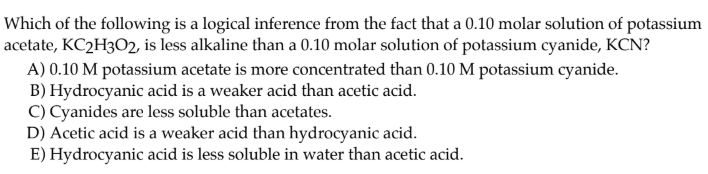# Which of the following is a logical inference from the fact that a 0.10 molar solution of potassium acetate, KC2H3O2, is less alkaline than a 0.10 molar solution of potassium cyanide, KCN? A) 0.10 M potassium acetate is more concentrated than 0.10 M potassium cyanide. B) Hydrocyanic acid is a weaker acid than acetic acid. C) Cyanides are less soluble than acetates. D) Acetic acid is a weaker acid than hydrocyanic acid. E) Hydrocyanic acid is less soluble in water than acetic acid.